# The Exploding Tree Function

## The Tree Transformation Rule

Consider a binary tree T of the form: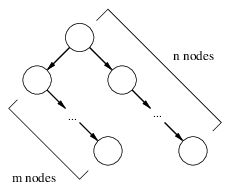where the left subtree is a linear right-branching chain of m nodes and the right subtree is a linear right-branching chain of n nodes, for some m and n. We shall call T a double right-branching chain of m left nodes and n right nodes.

Given some constant p and a double right-branching chain T of m left nodes and n right nodes where m>0 and n>0, we perform the following transformation:

1. Replace T with a right-branching chain of length (n+p);

2. For each of the (n+p) nodes in the new subtree, attach a right-branching chain of length (m-1) as its left child.

The result of this transformation looks like this: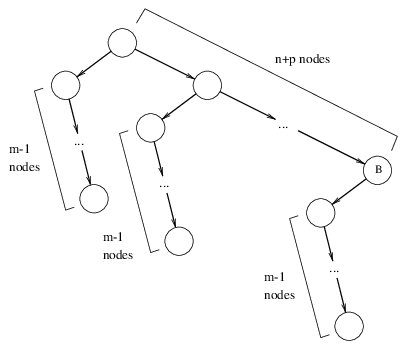We shall call a binary tree of this form a right-branching comb (or simply, a comb) of spinal length (n+p) and tooth length (m-1). We shall call the subtree rooted at the node marked B the rightmost tooth.

## The Linearization Theorem

We intend to show that (1) any binary tree T is either already a linear right-branching chain, or contains at least one subtree which is a double right-branching chain; and (2) by repeated applications of the tree transformation rule, T can be reduced into a linear right-branching chain. In other words, the transformation we defined above is a way of reducing any given binary tree into a linear right-branching chain of nodes. In the next section, we will show some other interesting properties of this transformation.

Before we prove the main theorem, however, we will need the following lemma:

Lemma 1. Given a double right-branching chain T of m left nodes and n right nodes, repeated applications of the tree transformation rule will eventually result in a linear right-branching chain.

Proof. We proceed by induction on m.

Base cases. For m=0, T is already a linear right-branching chain, so the lemma is vacuously true in this case. For m=1, we notice that m-1=0, and therefore, the left subtrees of the new (n+p) nodes are all empty—that is to say, the resulting tree is actually just a linear right-branching chain of (n+p) nodes.

Inductive case. Assume that any double right-branching chain of m-1 left nodes can be transformed into a linear right-branching chain. Let T be a double right-branching chain with m left nodes and n right nodes. We can apply the tree transformation rule to obtain a comb C of spinal length (n+p) and tooth length (m-1). Observe that the rightmost tooth of C is a double right-branching chain of (m-1) left nodes and 1 right node. By inductive hypothesis, this rightmost tooth can be transformed into a linear right-branching chain. Therefore, T becomes: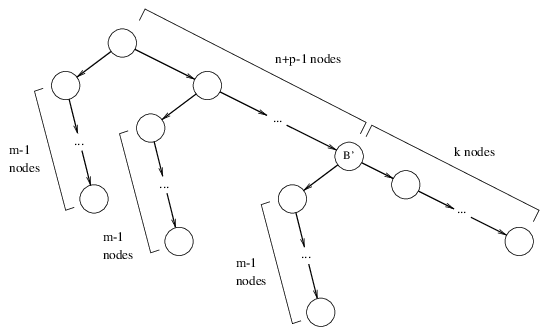for some number k. Note that we have decreased the number of teeth in the original comb by 1.

Now note that the subtree rooted at B' is a double right-branching chain with m left nodes and k right nodes. So we can apply our inductive hypothesis again to remove another tooth from the comb (and increasing the length of the right-branching chain to k', for some k'). Since there are (n+p) teeth in the original comb, all of which have length (m-1), we can apply our inductive hypothesis (n+p) times, and thus reduce the entire comb into a linear right-branching chain. QED.

Now we are ready to prove the main theorem.

Theorem 2 (Linearization Theorem). By repeatedly applying the tree transformation rule to any binary tree T, we can transform T into a linear right-branching chain.

Proof. To prove this theorem, we need to show that (1) any binary tree T that isn't already a linear right-branching chain always contains a subtree of the required form in order for the transformation to be applied; and (2) repeated applications of the transformation on T will eventually yield a linear right-branching chain. We proceed by induction on the number of nodes in a binary tree.

Base cases. A binary tree with 0 or 1 nodes is vacuously a right-branching chain of 0 or 1 nodes, respectively.

Inductive case. Suppose all binary trees of n nodes or less can be transformed into a right-branching chain. Now let T be a tree with n+1 nodes, where n≥1. A tree of n+1 nodes is simply a tree with n nodes, plus one more node N attached either as a left child or a right child of some parent node P.

Case A1: N is a left child: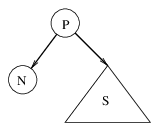Since the subtree S has at most n-1 nodes, by inductive hypothesis it can be transformed into a right-branching chain. Hence, the subtree rooted at P becomes: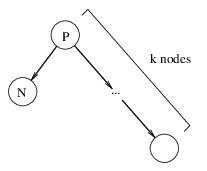for some number k. Since this is a double right-branching chain of 1 left node and k right nodes, we can apply the tree transformation rule, which yields a comb of spinal length n+m and tooth length of 0. But a comb with tooth length 0 is simply a linear right-branching chain.

Case A2: N is a right child: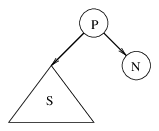Again, the subtree S has at most n-1 nodes, so by inductive hypothesis, it can be transformed into a right-branching chain: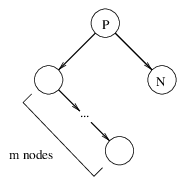for some number m. By Lemma 1, this subtree can be transformed into a linear right-branching chain.

Hence, the subtree rooted at P can be transformed into a linear right-branching chain. Now, there are 3 possible cases:

Case B1: P is the root of the T. In this case, we have completed the proof.

Case B2: P is the right child of some parent node Q. Since the left subtree of Q has less than n nodes, by inductive hypothesis it can be transformed into a linear right-branching chain. Then, by Lemma 1, the subtree rooted at Q can be transformed into a linear right-branching chain as well.

Case B3: P is the left child of some parent node Q. Since the right subtree of Q has less than n nodes, by inductive hypothesis it can be transformed into a linear right-branching chain. Then, by Lemma 1, the subtree rooted at Q can be transformed into a linear right-branching chain as well.

For cases B2 and B3, we can repeat the same argument to Q: if it is the root node, we are done; otherwise, we examine the tree rooted at Q's parent, and repeat the same argument. We can recursively apply this to each ancestor of Q until we reach the root node, at which time we apply case B1 to show that the entire tree can be reduced to a linear right-branching chain. QED.

## The Tree Linearizing Function

Now we shall define the Tree Linearizing Function, L(T,m), to be the length of the right-branching chain resulting from repeated applications of the tree transformation rule to a given binary tree T, with the constant p set to m.

The power of this function may not be immediately obvious, until we consider a few examples.

### Example 0

First, if T is a linear right-branching chain, then L(T,m) is simply the length of the chain. This is not very interesting.

### Example 1

Next, consider a left-branching chain of 2 nodes, which is equivalent to a double right-branching chain with 1 right node and 1 left node: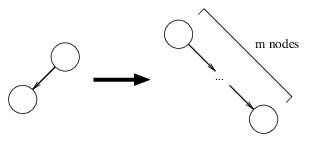By the tree transformation rule, this is transformed into a chain of length m. Hence, L(T,m)=m.

### Example 2

Now consider a double right-branching chain with 1 right node and 2 left nodes: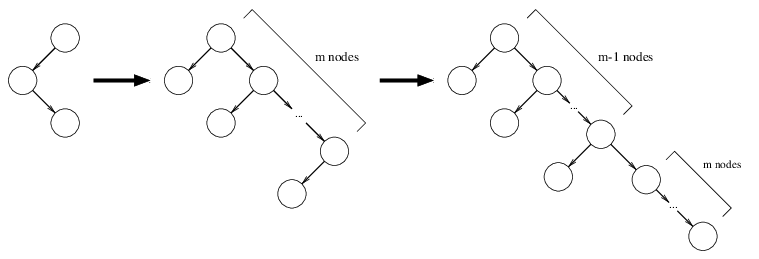The first application of the tree transformation rule yields a comb of spinal length m and tooth length 1. We may apply the transformation again to the rightmost tooth of this comb, which creates a linear right-branching chain of m nodes. Applying the transformation to the next rightmost tooth adds another m nodes to the chain. We can apply the transformation for each of the m teeth, and each time we increase the length of the linear chain by m. Hence, the final resulting chain has m⋅m=m2 nodes. Therefore, L(T,m)=m2.

### Example 3

Now consider the following double right-branching chain of 1 right node and 3 left nodes: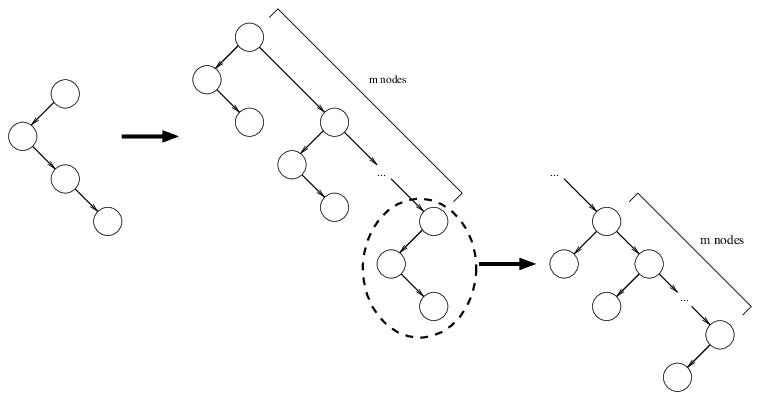The first application of the transformation rule yields a comb of spinal length m and tooth length 2. Applying the rule to the rightmost tooth yields a comb of spinal length m and tooth length 1. From the previous example, we know that this sub-comb transforms into a linear chain of length m2. Now, applying the transformation to the next rightmost tooth of the original comb yields a sub-comb of spinal length m2 and tooth length 1. This sub-comb transforms to a chain of length (m2)2=m4. As can be seen, each tooth of original comb squares the length of the linear chain. Since there are m teeth, the final chain will have length m2m. Therefore, L(T,m)=m2m.

### Example 4

Next, a double chain with 4 left nodes: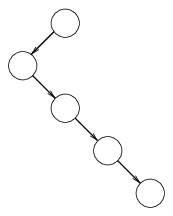The first application of the transformation rule yields a comb of spinal length m and tooth length 3. Applying the rule to the rightmost tooth yields a comb of spinal length m and tooth length 2. From the previous example, we know that this transforms to a chain of length m2m. Applying the transformation to the next rightmost tooth of the original comb yields a sub-comb of spinal length m2m and tooth length 2. This in turn transforms into a chain of length (m2m)2m2m + m2m = m2m2m+1 + m2m. For the sake of clarity, let's call this number C1. Transforming the next rightmost tooth of the original comb yields a sub-comb of spinal length C1 and tooth length 2. This in turn adds C12(C1) to the length of the chain, yielding C2 = C12(C1) + C1. In general, Ci+1 = Ci2(Ci) + Ci. Hence, each tooth of the original comb increases the height of the dominant term, the exponential tower, by 2. Therefore, the value of L(T,m) must be on the order of magnitude of an exponential tower of height 2m. Using Knuth's up-arrow notation, this is on the order of magnitude of m↑↑(2m).

### Larger left-branching trees

We can proceed to a double chain of 5 left nodes, but the length of the resulting chain cannot be adequately expressed by conventional notation. Using Knuth's up-arrow notation, it is on the order of m↑↑↑m.

This is, in fact, only a glimpse into the true power of L(T,m). Consider, for example, a 3-node linear left-branching chain: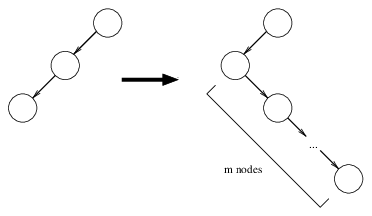The first application of the transformation rule yields a comb of spinal length 1 and tooth length m. Fully expanding this tree will result in a linear right-branching chain with a length on the order of m up-arrows in Knuth's notation (m↑mm, using the indexed Knuth up-arrow notation). In other words, it is on the order of the Ackermann function.

Adding just one right child to the leaf node of the 3-node left-branching chain results in a tree that, when fully expanded, far transcends Knuth's up-arrow notation. To get an idea of the immensity of the resulting chain, note that after the first application of the transformation rule, we have a comb of spinal length m and tooth length 2. Expanding rightmost tooth yields a sub-comb of spinal length m and tooth length (m-1). In other words, each tooth of this sub-comb is the equivalent of an Ackermann function; all of them combined is the equivalent of composing the Ackermann function with itself m times. But this is only one tooth of the original comb. The next tooth composes the m-times-composed Ackermann function with itself m times, and the next tooth composes the resulting function with itself m times, and so on. This process is repeated m times.

Keep in mind that the above results just from adding a single right child to the leaf of the 3-node left-branching chain. Adding more right children to the leaf results in the equivalent of functions quite possibly in the upper reaches of Bower's notation. Instead of adding just a right-branching chain of nodes, one could, of course, add a right subtree, such as another left-branching chain of 3 nodes.

But we have only barely begun to explore the power of L(T,m). What if we set T to a left-branching chain of four nodes? Or perhaps five nodes?

## The Exploding Tree Function

Now we shall define the Exploding Tree Function, E(n). Let the binary tree λn be the linear left-branching chain of n nodes. Then, E(n)=L(λn,n).

In other words, E(n) is the length of the right-branching chain produced by repeatedly applying the tree transformation rule to a left-branching chain of length n.

## Credits

Thanks to Rob Munafo, whose Large Numbers page inspired me to research the subject of large numbers in the first place.

The name of this page (and of E(n)) was inspired by Jonathan Bowers' Exploding Array Function, which motivated me to consider the use of fast-growing functions to generate large numbers. (Note: after a more detailed re-analysis of Bowers' Exploding Array Function, it has been found that E(n) actually only attains to the power of 5-element linear arrays. Bowers' system far transcends E(n) even just with linear arrays alone; with larger structures, it is in another league altogether.)

The definition of E(n) was inspired by the transfinite ordinals of the Veblen hierarchy and the Feferman-Schütte ordinal, Γ0.

Last updated 06 Feb 2018.